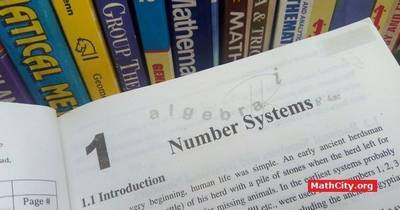# Chapter 01: Number SystemNotes (Solutions) of Chapter 01: Number System, Textbook of Algebra and Trigonometry Class XI (Mathematics FSc Part 1 or HSSC-I), Punjab Text Book Board, Lahore.

• Rational numbers and irrational numbers
• Properties of real numbers
• Complex numbers
• Operation on complex numbers
• Complex numbers as ordered pairs of real numbers
• Properties of the fundamental operations on complex numbers
• A special subset of $\mathbb{C}$
• The real line
• Real plane or coordinate plane
• Geometrical representation of complex numbers, the complex plane
• To find real and imaginary parts of (i) $(x+iy)^n$ (ii) $\left(\frac{x_1+iy_1}{x_2+iy_2}\right)^n, x_2+iy_2\neq 0$
Did you know $\sqrt{-1}=i$ is misleading?
The square root of complex number has many values with two distinct values. $\sqrt{-1}$ has two distinct values $i$ and $-i$. This fact can be verified by taking square of $i$ and $-i$, which give the same answer $-1$. It is different to say $i^2=-1$ and $\sqrt{-1}=i$ unless we define $\sqrt{-1}$ as principal square root of complex number. (see detail answer here)

The following short questions of this chapter was send by Mr. Akhtar Abbas.